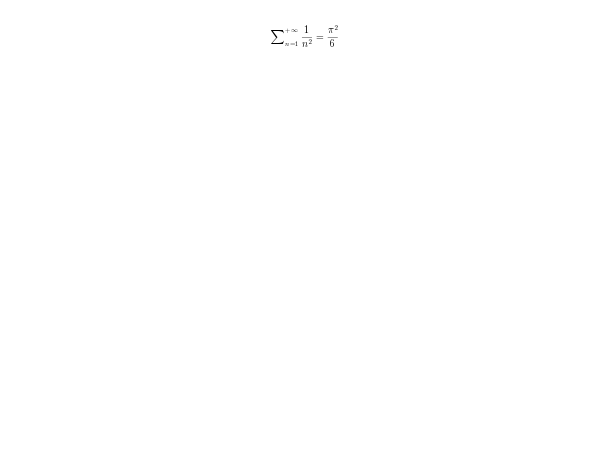Scilab Home page | Wiki | Bug tracker | Forge | Mailing list archives | ATOMS | File exchange
Change language to: English - Français - Português - 日本語 -

See the recommended documentation of this function

Справка Scilab >> Графики > text > Math rendering in Scilab graphics

# Math rendering in Scilab graphics

Display mathematical equations in Scilab graphics through the LaTeX or MathML languages.

### Usage

Starting from Scilab 5.2, it is possible to write LaTeX or MathML expression.

LaTeX texts must start and end by $(dollar symbol) while MathML texts must start by < and end by > and being syntactically valid. On the first use (these libraries are loading on the fly only when needed), note that the MathML engine is slower to load than LaTeX. // Example with LaTeX / MathML ticks: plot2d(); a=gca(); mathml="<mrow>;<mfrac><mrow><mi>d</mi><mi>y</mi></mrow><mrow><mi>d</mi><mi>x</mi></mrow></mfrac><mo>=</mo>.. <mfrac><mn>1</mn><msup><mi>y</mi><mn>2</mn></msup></mfrac></mrow>"; // LaTeX and MathML mixed expression a.x_ticks = tlist(["ticks", "locations", "labels"], (0:6)', [mathml;"1";"$\sin(x)$";"3";"$\cos(a) - test$";"5";"6"]); ### LaTeX description The rendering engine is based on the Java library JLaTeXMath. JLaTeXMath is an implementation of the mathematic mode of LaTeX. All LaTeX base commands are handle (do not hesitate to submit a bug report if missing). Few TeX commands, like \over are supported. Since Scilab 5.2.1, greek, cyrillic and complete unicode for latin alphabets characters are handled in the Scilab graphicsxtitle('$\textstyle\sum_{n=1}^{+\infty}\frac1{n^2}=\frac{\pi^2}{6}$') xtitle('$\big(\bigg)$') xtitle('$\mbox{Vector field for }\ddot{\theta}=\sin\theta$') xtitle('$\JLaTeXMath\ \mathfrak{and}\ \mathtt{Scilab}$') xstring(0.5,0.5,"$\overbrace{привет\ мир} \underbrace{γειά\ κόσμο}$") JLaTeXMath provides several fonts with the commands \mathbb, \mathscr, \mathcal, \mathbf, \mathit, \mathsf, \mathtt, \mathfrak, \mathds, \mathrm, with their bold versions when they are available with the command \boldsymbol : xtitle('$\mathbb{SCILAB}\ \mathsf{or}\ \boldsymbol{\mathfrak{Scilab}}$') xtitle('$\mathscr{C}\mbox{ n''est pas }\boldsymbol{\mathcal{C}}$') Different LaTeX packages are available: amsmath, amssymb, stmaryrd, amsxtra and accents with some commands of graphics. Most of the commands of these packages are available (some of amsmath are missing for example). xtitle('$\sideset{_\alpha^\beta}{_\gamma^\delta}\prod$') xtitle('$\hat{\accentset{\star}{\hat h}}\undertilde{ABC}$') xtitle('$\begin{pmatrix}\mathfrak{a}&\alpha\\\mathbb{A}&\mathcal{A}\end{pmatrix}\mbox{ or }..
\begin{bmatrix}\mathfrak{a}&\alpha\\\mathbb{A}&\mathcal{A}\end{bmatrix}$') xstring(0.5,0.5,'$\left(\frac{\pi}{\sqrt{2}}\middle|\sqrt{\frac{1+\frac1x}{x}}\right\rangle$') xtitle('$\doublecup\ddag\fatbslash\lll\oplus\ovee\circledcirc\circlearrowright$') xtitle('$\rotatebox{180}{\boxed{\JLaTeXMath}}\ \reflectbox{\JLaTeXMath}$') xtitle('$\scalebox{0.6}{\sum_{n=1}^{+\infty}\frac1{n^2}=\frac{\pi^2}6}$') xtitle('$\fcolorbox{black}{Tan}{\JLaTeXMath}$') xtitle('$\textcolor{Magenta}{\mathfrak{Scilab}}\mbox{ and }\textcolor{Green}{\mathcal{SCILAB}}$') It is also possible to define new commands or new environments: xtitle('$\newcommand{\op}{\left(}\newcommand{\cp}{\right)} \op\frac12\cp$') xtitle('$\newcommand{\myfrac}{\frac{\mathfrak{#1}}{\mathcal{#2}}}\myfrac{A}{B}\$')

### MathML description

The MathML rendering is based on Jeuclid. Jeuclid is a MathML implementation which covers the whole specification. Therefor, all the MathML language is supported within Scilab.

Due to the size of the Jeuclid library, on the first use, it can take up to a few seconds to load. However, next uses are much faster.plot3d();
a=get("current_axes");
a.x_label.font_size= 5;
a.x_label.text="<mrow><mfrac><mrow><mn>1</mn></mrow><mrow><mn>2</mn></mrow></mfrac><mo></mo><mfenced open=""{"" close=""}""> ..
<mrow><mfrac><mrow><mfrac><mrow><mi>a</mi></mrow><mrow><mi>b</mi></mrow></mfrac></mrow><mrow><mfrac><mrow> ..
<mi>c</mi></mrow><mrow><mi>d</mi></mrow></mfrac></mrow></mfrac></mrow></mfenced></mrow>"